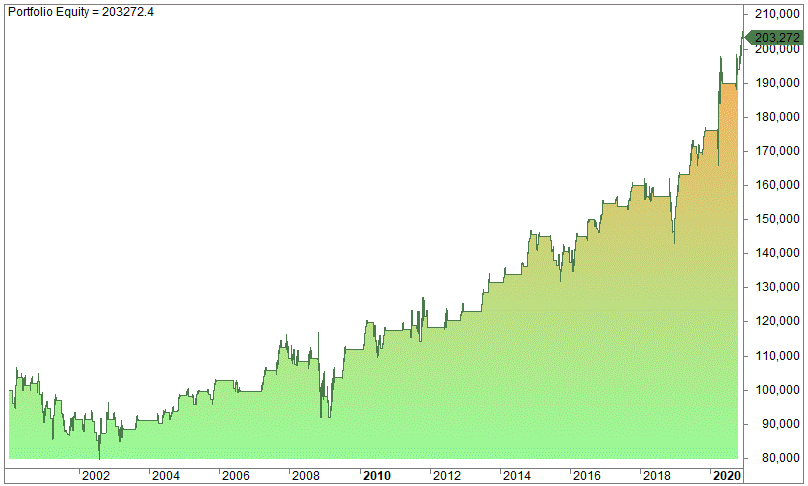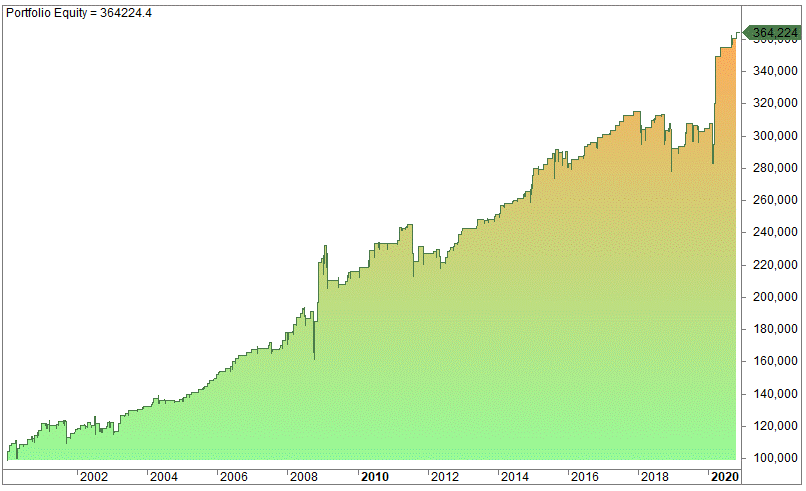# MACD Trading Strategy – Does The Indicator Work? (System Backtest and Explanation)

Last Updated on June 19, 2022 by Quantified Trading

Can we use the MACD indicator and the MACD histogram to make a profitable MACD trading strategy?

This article looks at the MACD-indicator, what it is, and how you can use it. Does the MACD indicator work? In which markets does it work best? Which time frame is best?

The MACD indicator is a useful tool and seems to work on stock indices. We also find the MACD useful for trading strategies.

The MACD was first mentioned as long back as in the 1970s. MACD is an abbreviation for moving average convergence/divergence. It’s simply an indicator that consists of two moving averages and how these two lines converge and diverge from each other.

If you find this article useful, you might want to have a look at our landing pages for a lot of other trading strategies and edges:

## How to calculate the MACD:

Let’s start by looking at how to calculate the MACD indicator, which is done in two steps. We include a third step, which is calculating the MACD histogram.

The first step is to calculate the MACD line:

### The fast MACD line

The MACD line is also called the fast line. This is the 12-day exponential moving average deducted from the 26-day exponential average. In Amibroker, the code looks like this:

EMA(c,12) – EMA(c,26)

The fast MACD line is, in other words, the difference between the short and the long moving average. The line is often referred to as the MACD line.

### The slow MACD line/the signal line

The second step is to calculate the slow line which is the 9-day exponential moving average of the fast MACD line. This is called the signal line. The lagging signal line is often used as a reversal signal, something we’ll explain later.

### The MACD histogram

This is a deviation from the original MACD indicator, which was developed later, we believe by Alexander Elder in the book called Trading For A Living. The histogram is the fast line subtracted the slower signal line. This relationship is quite important, and back in 2012, we published an article about developing a strategy based on the MACD-histogram.

### Why the parameters of 12, 26, and 9?

In all trading software, books, seminars, etc., the default settings are 12, 26, and 9 days. Why is that? We assume only the inventor of the MACD, Gerald Appel, can answer that. There is, of course, nothing stopping you from changing the parameters. However, the above settings have somehow been “stuck” with the indicator.

The chart below shows how the MACD oscillates back and forth:The red line is the MACD line (the fast line), while the blue line is the slow signal line. The bars oscillating around zero is the MACD-histogram.

## How does the MACD indicator work?

The MACD is an oscillating indicator that can capture both momentum, reversal, and trend. As always, there is no definitive answer on how to use the MACD. Most likely, you will find the best use not mentioned in any books or articles!

## Which MACD setting is best? What is the best time frame for the MACD?

You have to play around with the values. The default values are just suggestions, and you need to play around with the values in order to see if you spot any patterns.

For example, the market indices move fast up and down, and you are probably better off if you use a shorter time frame.

Again, there is no definitive answer, and you must test yourself what works and what doesn’t. Be careful to generalize to other markets. No indicator works well on all markets! Something that works on the S&P 500 doesn’t necessarily work on the gold price.

Let’s make a MACD trading strategy on the S&P 500.

### Buy when the fast line crosses above the slow signal line

The above criterion is taken from Alexander Elder’s Trading For A Living. Elder didn’t test the strategy, even though this strategy is easily tested.

1. Buy on the close when the fast MACD line crosses above the slow signal line.
2. Sell on the close when the slow signal line crosses above the fast MACD line.

This is all there is to it. On the S&P 500, we get this equity curve for the MACD trading strategy:The CAGR is 4.19%, the average gain per trade is 0.44%, the profit factor is 1.65, and the maximum drawdown is 30%.

The code in Amibroker is like this:

Fast = EMA(C,12) – EMA(C,26);
Slow = EMA(Fast,9);

Sell = Cross(Slow, Fast);
sellPrice = Close ;

The strategy is certainly untradeable. If we start optimizing, what are the best parameters?

To make an optimization we need to rewrite the code:

Opt1 = Optimize(“ShortEMA”,2,2,15,1);
Opt2 = Optimize(“LongEMA”,20,6,25,1);
Opt3 = Optimize(“Slow”,5,5,15,1);

Fast = EMA(C,Opt1) – EMA(C,Opt2);
Slow = EMA(Fast,Opt3);

Sell = Cross(Slow, Fast);
sellPrice = Close ;

This gives 3080 optimization steps. Amibroker is lightning fast and the optimization is done in 13 seconds. The results indicate the best parameters are somewhat in the vicinity of the default settings.

Let’s change the strategy to include a new variable but keep the same parameters in the MACD crossover:

We have reasons to suspect a MACD crossover is more bullish if it happens when the slow line is in negative territory. Is this correct? Let’s test by adding a new variable (in bold):

Fast = EMA(C,12) – EMA(C,26);
Slow = EMA(Fast,9);

Buy = Cross(Fast, Slow) and Slow<0;
Sell = Cross(Slow, Fast);
sellPrice = Close ;

When the slow signal line is below zero when the crossover happens, we slightly improve the results, but the number of trades is more than halved:The CAGR is 3.44%, the average gain per trade is 0.79%, the profit factor is 1.72, and the maximum drawdown is 25%.

If we add a long-term trend filter, for example, a 200-day average, the results improve.

### MACD as overbought and oversold trading strategy

The MACD can additionally help us to find overbought and oversold conditions. We have already mentioned the MACD-histogram, and this indicator shows the difference between the fast and the slow signal line. When the distance is increasing, it means the speed of the fast line is picking up more than the slow signal line.

Let’s try a new twist of the MACD by using the MACD-histogram. For simplicity, we use the same strategy as in this article about MACD histogram strategy. The requirements are the following:

1. The MACD histogram bar must have fallen 4 days in a row.
2. The fourth latest bar must have been below zero.
3. The current close of the ETF must be lower than the day before.
4. If the above three criteria are true, then enter at the close.
5. Exit on the close when today’s close is higher than yesterday’s high.

The Amibroker code is like this:

Fast = EMA(C,12) – EMA(C,26);
Slow = EMA(Fast,9);
Histogram = Fast – slow;

Buy= C<Ref(C,-1) AND Ref(Histogram,-4) < 0 AND Ref(Histogram,-4) > Ref(Histogram,-3) AND Ref(Histogram,-3) > Ref(Histogram,-2) AND Ref(Histogram,-2) > Ref(Histogram,-1) AND Ref(Histogram,-1) > Histogram;
Sell = Close > Ref(H,-1);
sellPrice = Close ;

Here is an example of such a trade signal (using 3, 10, and 5-day lookback period):By using 12, 26, and 9-day lookback periods, we get the following equity curve on the S&P 500 (compounded):The CAGR is 4.79%, the average gain per trade is 0.95%, the profit factor is 4.22, and the maximum drawdown is 16%. This shows some promise, and it works pretty well on several other ETFs as well.

## Combining MACD Histogram and the RSI indicator into a strategy

In a previous article, we covered how the RSI indicator works. The RSI indicator has proven to be a useful mean revertive indicator, and it might add value combined with the MACD histogram. Let’s add a “slow histogram” and combine that with a two-day RSI. The idea is that we only buy on a low RSI but it must be combined with a low histogram reading. This is the code in Amibroker:

Fast= EMA(C,12) – EMA(C,26);
Slow= EMA(Fast,9);
Histogram= Fast – Slow;
HistogramSlow= MA(Histogram,5);

Buy= RSI(2) < 10 AND HistogramSlow < Ref(HistogramSlow,-5) ;
Sell= Close > Ref(H,-1);
sellPrice= Close ;

The compounded equity curve for the S&P 500 looks like this:The CAGR is 6.36%, the average gain per trade is 0.76%, the profit factor is 2.45, and the maximum drawdown is 16%. Compared to the MACD histogram strategy it has more trades and works better compared to only trading the two-day RSI without any other criteria.

We have covered many other indicators and strategies in previous articles. For a full list, please see this link:

As with all other indicators, the MACD is a lagging indicator. The moving average crossover most likely has limited use as a stand-alone trading signal, but the MACD histogram trading strategy shows some real promise. However, the MACD-histogram is a mean reversion indicator, and there are probably other and better indicators for that, like for example, the RSI indicator.

## Similar Posts

•Erik Tsang says:

I tested out the code from 1999 to 2020, seems the MACD strategy does not work at all before 2000. the drawdown is 50%. I guess the market regime changed.

•Oddmund Groette says:

Hi Erik,
Thanks for the testing.
Oddmund

•Ben says:

Hey,

I’m curious as to why you use sellPrice= Close ; and buyPrice= Close; as they are not really realistic imo (unless you have a trick for that?).

If you use a more realistic next day buy and sell prices of AvgPrice = (O+H+L+C)/4; your results will look a lot different (and unfortunately a lot worse).

Cheers,

•Oddmund Groette says:

Hi Ben,
Your question is relevant. You have three options to trade a signal: on the close, after the close (after hours), or next day.

I prefer to enter on the close. It’s not really on the close, but I enter ten seconds before the exchange closes. The results are better by entering on the same bar than the next bar (for example of the open).

You’re right, it will be a lot worse by using next day. This is the sad reality. I’m interested in doing what works and thus I enter just before the close:) This is the power of automation: the computer does it for you.

Regards,
O.G.